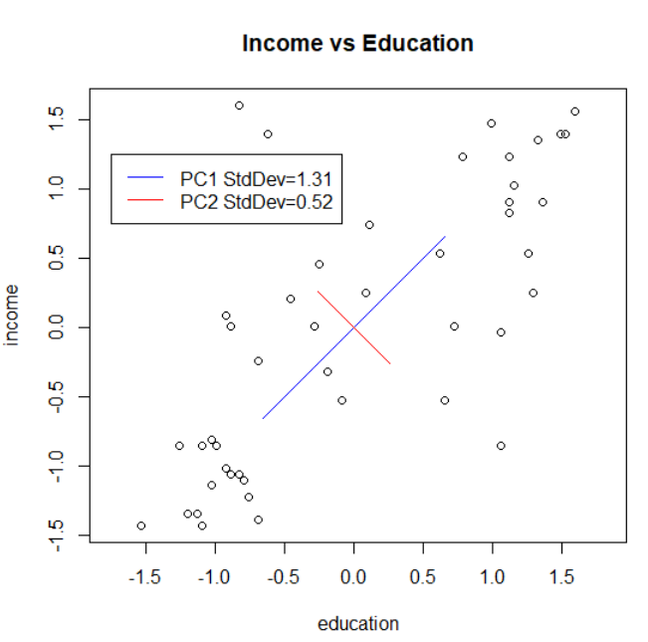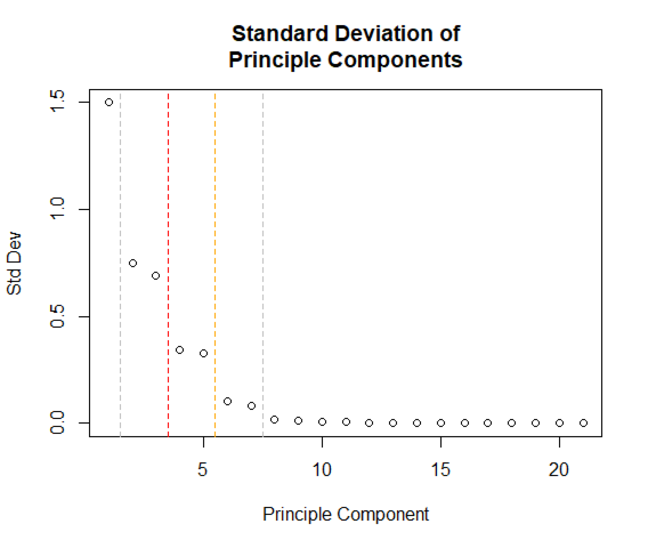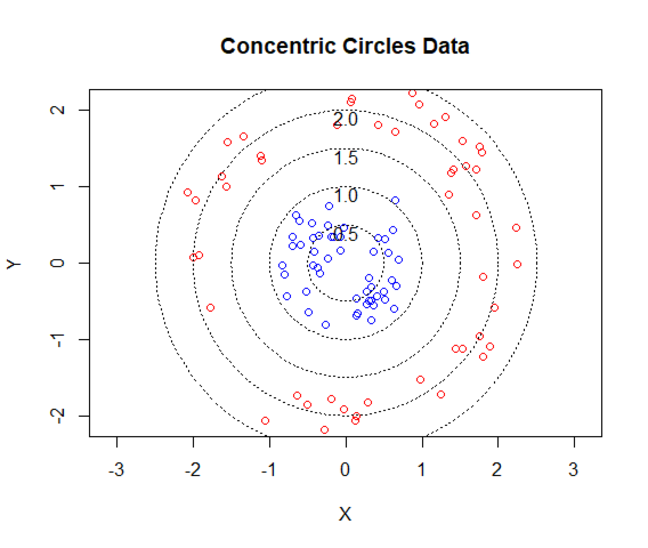## Want to keep learning?

This content is taken from the The Open University & Persontyle's online course, Advanced Machine Learning. Join the course to learn more.
4.5

# Principal Components Analysis

Principal components analysis (PCA) is a linear dimensionality reduction technique that will produce linear mixtures of the original input features from which a small subset of highly informative features can be easily extracted. It relies on the (typically reasonable) assumption that there is a strong correlation between variance and information, such that features that exhibit high variance are likely to provide a large amount of information. It is used for both supervised and unsupervised problems.

## PCA algorithm

Given a set of centered (normally) and scaled (essentially) real valued features, $$X$$, the PCA finds the principle components of the $$X^TX$$ matrix, and associated with each principle component a value indicating the amount of variance or standard deviation of the data in the given direction. Mathematically, these principle components are the eigen-vectors of the $$X^TX$$ matrix, and the associated values are the associated eigen-values. The set of principle components form a new orthogonal basis for the original feature space that is a rotation of the original feature basis set.

The result is that we get an ordered list of vectors (the components), each of which is the direction of maximal variance orthogonal to the preceeding vectors.The above image provides a visual example. The principal component directions are plotted on the original data, with length corresponding to the standard deviation of the data in the given direction.

We can now obtain the coordinates of the data points in terms of the principal components, thus giving us a new, transformed set of features. Each of these principal component features being a linear combination of the original features.

To see why this is useful, let us make the assumption that information is likely to exist in directions where there is significant variance. This assumption is plausible, but not infallible - so we should always try alternative approaches as well. Given the assumption, it is simple to make a principled decision about which principle component features are valuable and which are not: We simple look at the amount of variance the data has in for each. Since the principal components are ordered by the amount of variance, this means that we will take the first $$n$$ principal component features, and need only decide on the value of $$n$$.

To decide on this value, we typically look at the variance/standard deviation in each direction. We will likely see something like this:Here the red and orange divisions (taking principal components to the left of the dashed line) look most promising, but they gray divisions might also be interesting.

It is possible to automate the decision of which principal components to use. Popular approaches are to look for large reductions in the amounts of variance, to look for the ‘elbow’ of the graph (where the line connecting the dots crosses through 45 degrees, though it can do this multiple times, like in the example above), or where the variance drops below some specified level (either absolute, or as a percentage of total variance).

Note using that the complete set of principal components is not useful. They form nothing more than a rotation of the original data. When you data contains both real and discrete features, you can perform PCA on the real features and use the resulting principal components with the original discrete features.

## Non-linear Extensions

PCA is a linear feature transformation: The new features are linear combinations of the original features. This can work well with some data sets, and poorly with others. For example, in the diagram below we would be much better off transforming our data such that we work with the distance a point is from the center of the circles that any subset of axises from a rotation of the data.Projecting onto the distance from the center of the circle is an example using a non-linear feature transformation (in this case, projecting onto a radial basis function). Non-linear feature transformations, like projecting the data onto a curve, can be powerful. Indeed, doing so formed a part of all the advanced supervised learning techniques we covered. Doing so manually, as part of preprocessing, means that you do not need to restrict yourself to the sort of non-linear transformations that are performed as part of the modeling algorithms you are working with.

There are a huge number of a non-linear feature transformation approaches available, and research into such transformations continues apace. One case is known as kernel-PCA, and this make use of the fact that feature vectors occur in PCA only inside inner-products (the $$X^TX$$ calculation) and therefore we are able to perform the kernel trick on PCA to obtain a non-linear extension: Instead of the eigenvalues of the $$X^TX$$ matrix, we seek the eigenvalues of the kernel matrix. As with all these feature transformations, kernel PCA can sometimes help a lot, and sometimes not at all. The best approach for most problems is to use them and see!